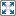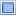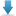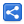# Java - Iterator vs Spliterator

[Last Updated: Apr 21, 2017]

The Collection interface extends Iterable, that means all collection implementations are iterable and we can iterate over collections elements like this:

``` List<String> list = Arrays.asList("Apple", "Banana", "Orange");
for (Iterator<String> i = list.iterator(); i.hasNext(); ){
System.out.println(i.next());
}
```

Output:

```Apple
Banana
Orange
```

We can achieve the same by using Java 5 enhanced for loop:

``` List<String> list = Arrays.asList("Apple", "Banana", "Orange");
for (String s : list) {
System.out.println(s);
}```

## Iterator interface

```package java.util;

import java.util.function.Consumer;

public interface Iterator<E> {

boolean hasNext();

E next();

default void remove() {
throw new UnsupportedOperationException("remove");
}

default void forEachRemaining(Consumer<? super E> action) {
Objects.requireNonNull(action);
while (hasNext())
action.accept(next());
}
}
```

Java 8 added two new default methods in Iterator class: `remove()` which by default is not supported, it is supported by all JDK collection implementations except for fixed-size collections e.g. the one create from `Arrays.asList`.

Another new method in iterator interface is: `forEachRemaining` which by default performs the given action for each remaining element:

``` List<String> list = Arrays.asList("Apple", "Banana", "Orange");
Iterator<String> i = list.iterator();
i.next();
i.forEachRemaining(System.out::println);
```

Output:

```Banana
Orange
```

## Iterable interface

Let's take a step back and have a look at Iterable interface which is implemented by all collections:

```package java.lang;

import java.util.Iterator;
import java.util.Objects;
import java.util.Spliterator;
import java.util.Spliterators;
import java.util.function.Consumer;

public interface Iterable<T> {

Iterator<T> iterator();

default void forEach(Consumer<? super T> action) {
Objects.requireNonNull(action);
for (T t : this) {
action.accept(t);
}
}

default Spliterator<T> spliterator() {
return Spliterators.spliteratorUnknownSize(iterator(), 0);
}
}
```

Java 8 added two new default methods to the Iterable interface. One of them is `foreach` which uses enhanced for loop by default. This method was added to take advantage of lambda expression. Now our above example can be rewritten as:

``` List<String> list = Arrays.asList("Apple", "Banana", "Orange");
list.forEach(System.out::println);
```

Another new method in Iterable interface is `spliterator()` which creates an instance of Spliterator. The default implementation creates an early-binding spliterator from the iterable's Iterator instance. We will see what is early-binding spliterator shortly. First let's understand what Spliterator is itself.

## Spliterator interface

Spliterator can be used to split given element set into multiple sets so that we can perform some kind of operations/calculations on each set in different threads independently, possibly taking advantage of parallelism. It is designed as parallel analogue of Iterator. Other than collections, the source of elements covered by a Spliterator could be, for example, an array, an IO channel, or a generator function.

Here's the interface snippet:

```package java.util;

import java.util.function.Consumer;
import java.util.function.DoubleConsumer;
import java.util.function.IntConsumer;
import java.util.function.LongConsumer;

public interface Spliterator<T> {

default void forEachRemaining(Consumer<? super T> action) {
}

Spliterator<T> trySplit();

long estimateSize();

default long getExactSizeIfKnown() {
return (characteristics() & SIZED) == 0 ? -1L : estimateSize();
}

int characteristics();

default boolean hasCharacteristics(int characteristics) {
return (characteristics() & characteristics) == characteristics;
}

default Comparator<? super T> getComparator() {
throw new IllegalStateException();
}

public static final int ORDERED    = 0x00000010;
public static final int DISTINCT   = 0x00000001;
public static final int SORTED     = 0x00000004;
public static final int SIZED      = 0x00000040;
public static final int NONNULL    = 0x00000100;
public static final int IMMUTABLE  = 0x00000400;
public static final int CONCURRENT = 0x00001000;
public static final int SUBSIZED = 0x00004000;

/*rest are some methods and nested implementations
specifically used by java.util.stream.*/
}

```

Let's go through each method quickly

With tryAdvance(), we can traverse underlying elements one by one (just like Iterator.next()). If a remaining element exists, this method performs the consumer action on it, returning true; else returns false.

For sequential bulk traversal we can use forEachRemaining():

``` List<String> list = Arrays.asList("Apple", "Banana", "Orange");
Spliterator<String> s = list.spliterator();
System.out.println(" --- bulk traversal");
s.forEachRemaining(System.out::println);

System.out.println("Element exists: "+b);
```

Output:

```Apple
--- bulk traversal
Banana
Orange
Element exists: false```

#### Spliterator<T> trySplit()

Splits this spliterator into two and returns the new one:

```  List<String> list = Arrays.asList("Apple", "Banana", "Orange");

Spliterator<String> s = list.spliterator();
Spliterator<String> s1 = s.trySplit();

s.forEachRemaining(System.out::println);
System.out.println("-- traversing the other half of the spliterator --- ");
s1.forEachRemaining(System.out::println);
```

Output:

```Banana
Orange
-- traversing the other half of the spliterator ---
Apple
```

An ideal trySplit method should divide its elements exactly in half, allowing balanced parallel computation.

The splitting process is termed as 'partitioning' or 'decomposition' as well.

#### characteristics() and hasCharacteristics()

A spliterator can expose it's implementation characteristics as a set of constants defined in the same interface.

```    public static void main (String[] args) {
List<String> list = new ArrayList<>();

Spliterator<String> s = list.spliterator();

if(s.hasCharacteristics(Spliterator.ORDERED)){
System.out.println("ORDERED");
}
if(s.hasCharacteristics(Spliterator.DISTINCT)){
System.out.println("DISTINCT");
}
if(s.hasCharacteristics(Spliterator.SORTED)){
System.out.println("SORTED");
}
if(s.hasCharacteristics(Spliterator.SIZED)){
System.out.println("SIZED");
}

if(s.hasCharacteristics(Spliterator.CONCURRENT)){
System.out.println("CONCURRENT");
}
if(s.hasCharacteristics(Spliterator.IMMUTABLE)){
System.out.println("IMMUTABLE");
}
if(s.hasCharacteristics(Spliterator.NONNULL)){
System.out.println("NONNULL");
}
if(s.hasCharacteristics(Spliterator.SUBSIZED)){
System.out.println("SUBSIZED");
}
}
```

Output:

```ORDERED
SIZED
SUBSIZED
```

Replacing above collection with HashSet will give this output:

```DISTINCT
SIZED
```

These characteristics can be used to control client side logic. For example a Java 8 stream pipeline makes it's internal decisions based on these characteristics. The low-level stream factory class StreamSupport takes these characteristics or the spliterator instance while creating the stream for this data source. For example:

```static <T> Stream<T>  stream(Spliterator<T> spliterator, boolean parallel)

static <T> Stream<T>  stream(Supplier<? extends Spliterator<T>> supplier,
int characteristics, boolean parallel)
```

#### estimateSize() and getExactSizeIfKnown()

estimateSize() returns the estimated number of elements that can be traversed at a given point. It can return Long.MAX_VALUE if the number of elements are infinite, unknown, or too expensive to compute.

The default method getExactSizeIfKnown() is a convenience method, as seen in the above interface definition. It returns estimateSize() if this Spliterator has a characteristic of SIZED, else returns -1.

Here's a usage example of these two methods:

```  public static void main (String[] args) {
List<String> list = Arrays.asList("Apple", "Banana", "Orange");
Spliterator<String> s = list.spliterator();
System.out.println(s.estimateSize());
System.out.println(s.getExactSizeIfKnown());
}
```

Output:

```Apple
2
2
```

#### getComparator()

If this Spliterator's source has characteristic SORTED, this method returns the corresponding Comparator. If the source is SORTED in natural order, it returns null. Otherwise, if the source is not SORTED, throws IllegalStateException.

```  SortedSet<Test> set =
new TreeSet<>((o1, o2) ->
o1.str.compareTo(o2.str));
Spliterator<Test> s = set.spliterator();
System.out.println(s.getComparator());
System.out.println(set)
```

Output:

```com.logicbig.example.collection.SpliteratorComparatorExample\$\$Lambda\$1/990368553@6d311334
[Test{str='one'}, Test{str='two'}]
```

### Spliterator implementation for primitives

Spliterator interface defines couple of inner interfaces which are specialized spliterator for primitive values. These interfaces are `Spliterator.OfPrimitive`, `Spliterator.OfInt`, `Spliterator.OfLong` and `Spliterator.OfDouble`

From Java docs:

The subtype default implementations of tryAdvance(java.util.function.Consumer) and forEachRemaining(java.util.function.Consumer) box primitive values to instances of their corresponding wrapper class. Such boxing may undermine any performance advantages gained by using the primitive specializations. To avoid boxing, the corresponding primitive-based methods should be used. For example, Spliterator.OfInt.tryAdvance(java.util.function.IntConsumer) and Spliterator.OfInt.forEachRemaining(java.util.function.IntConsumer) should be used in preference to Spliterator.OfInt.tryAdvance(java.util.function.Consumer) and Spliterator.OfInt.forEachRemaining(java.util.function.Consumer)

Here's an example:

``` public static void main (String[] args) {
int[] ints = {3,4,6,7};
Spliterator.OfInt s = Arrays.spliterator(ints);
s.forEachRemaining((IntConsumer) System.out::println);
}
```

Output:

```3
4
6
7
```

## Spliterators class

Spliterators provides static methods for creating Spliterator. This class is useful for creating a standalone Spliterator (without any collection), we can also create streams from such standalone spliterators using StreamSupport class. This class is also useful for converting iterator to Spliterator.

As mentioned above, the default method Iterable#spliterator returns a Spliterator created from `Spliterators.spliteratorUnknownSize()`. This method of Spliterators creates a Spliterator using a given Iterator as the source of elements, with no initial size estimate.We also mentioned that the default implementation creates an early-binding spliterator. This kind of spliterator binds the source of elements at the point of construction, whereas late-binding Spliterator binds to the source of elements at the point of first traversal, first split, or first query for estimated size, rather than at the time the Spliterator is create.

Here's a Spliterators example, creating a Spliterator covering the elements of a given array:

```  public static void main (String[] args) {
Spliterator<String> s = Spliterators.spliterator(
new String[]{"one", "two"}, 0);
s.forEachRemaining(System.out::println);
}
```

Output

```one
two
```

Note that we could have also used Arrays.spliterator(Object[]) in our above example instead.

## Example Project

Dependencies and Technologies Used:

• JDK 1.8
• Maven 3.0.4

 Iterator Spliterator ExamplesSelect AllDownload• iterator-spliterator-examples
• src
• main
• java
• com
• logicbig
• example
• SpliteratorTrySplitExample.java

 Share